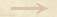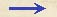Other Propositions 1/01-th-modified Other Proposition1/01-th-modifiedGlobal Velocity Theorem We find in Ms. Gal. 72 a proposition related to 1/01-th-01 that states the proportionality of spaces and times for motions with equal velocities in corresponding intervals. The proposition is stated (and proven) on folio 138v as follows: If there were an arbitrary number of spaces and others equal to them in number which, taken pairwise, have the same ratio and through which two movables move so that the motions (lationes) in arbitrary two spaces corresponding to each other are equal and uniform then as all preceding spaces are to all following ones so will be the times of all preceding motions to the times of all subsequent motions through the spaces.Other Propositions 1/01-th-modified Other Proposition1/01-th-modified